# Engineering Maths - Probability

>>>>>>>>Probability

Let A and B be two events defined as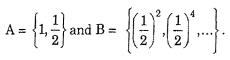• A

0• B

1• C• D

none of these• Option : A
• Explanation : A ∩ B = φ

P (A ∩ B) = 0

i.e.,  P(E1 ∪ E2 ∪ E3 .... ∪ En

= P(E1) +  P(E2) +....  + P(En)

• A

Law of total probability• B

Law of probability• C

Both (a) and (b)• D

None of these• A

963/1000• B

973/1000• C

983/1000• D

None of these• Option : B
• Explanation : S = [0, 1, 2, ....,9)
Suppose we want a particular integer "3" to be
chosen.
9
Probability of not choosing "3" is 9/10 (in one
chance)
Probability of not choosing 3 in all the three
chances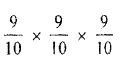Hence probability of choosing it in at least one
of the three chances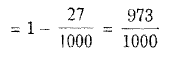• A

3/15• B

5/16• C

7/16• D

9/16• Option : B
• Explanation : The five digits can be arranged in 5! ways, out
of which 4! will begin with zero.

Total number of 5-figure numbers formed
= 5! - 4! = 96.
Those numbers formed will be divisible by 4
which will have two extreme right digits
divisible by 4,
i.e., numbers ending in 04, 12,20,24,32,40.
Now, numbers ending in 04 = 3! = 6,
numbers ending in 12 = 3! - 2! = 4,
numbers ending in 20 = 3! = 6,
numbers ending in 24 = 3! - 2! = 4,
numbers ending in 32 = 3! - 2! = 4,
and numbers ending in 40 = 3! = 6.
[Numbers having 12, 24, 32 in the extreme right
are (3! - 2!), since the numbers having zero on
t.he extreme left are to excluded.]
Total number of favourable ways
6 + 4 + 6 + 4 + 4 + 6 = 30
Hence,    required probability =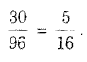• A

43/88• B

43/91• C

43/93• D

none of these• Option : B
• Explanation : Two balls out 14 can be drawn in 14C2 ways which is the total number of outcomes.

Two white balls out of 8 can he drawn in 8C2 ways

Probability of drawing 2 white balls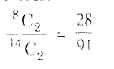Similarly 2 red balls out of 6 call be drawn in
6C2 ways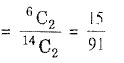Probability of drawing 2 red balls
Probability of drawing 2 balls of the same
colour (either both white or both red)Related Quiz.
Probability